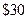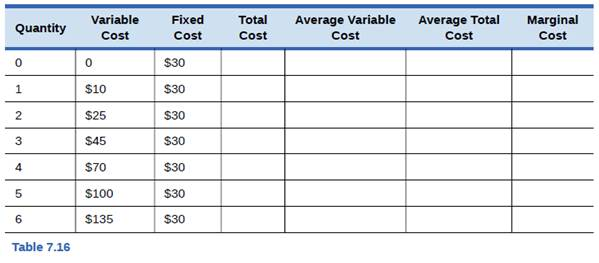Chapter 7, Problem 3SCQ### Principles of Economics 2e

2nd Edition
Steven A. Greenlaw; David Shapiro
ISBN: 9781947172364

#### Solutions

Chapter
Section### Principles of Economics 2e

2nd Edition
Steven A. Greenlaw; David Shapiro
ISBN: 9781947172364
Textbook Problem

# The WipeOut Ski Company manufactures skis for beginners. Fixed costs areFill in Table 7.16 for total cost, average variable test, average total cost, and marginal cost.To determine

The Total Cost, Average Variable Cost, Average Total Cost and Marginal Cost.

Explanation

Total Cost: Fixed Cost + Variable Cost

Average Variable Cost: Variable Cost/ Quantity

Average Total Cost: Total Cost/ Quantity

Marginal Cost: Total Cost1- Total Cost0

 Quantity- Variable Cost- I Fixed Cost-II Total Cost(I+II)-III Average Variable Cost(I/Quantity) Average Total Cost(III/Quantity) Marginal Cost(III)Qi-Q0 0 0 \$30 \$30(0+30) - - 1 \$10 \$30 \$40(10+30) \$10...

### Still sussing out bartleby?

Check out a sample textbook solution.

See a sample solution

#### The Solution to Your Study Problems

Bartleby provides explanations to thousands of textbook problems written by our experts, many with advanced degrees!

Get Started